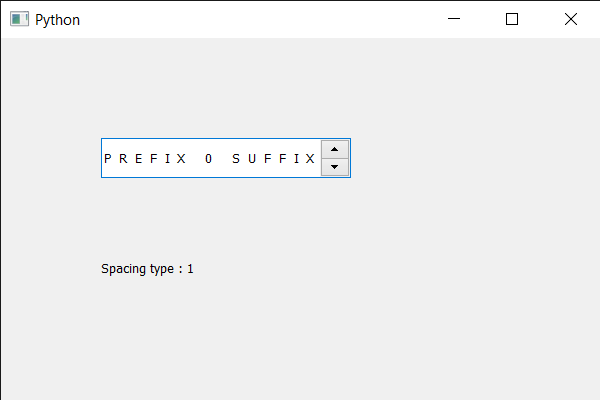# PyQt5 QSpinBox – Getting letter spacing type

• Difficulty Level : Basic
• Last Updated : 22 May, 2020

In this article we will see how we can get the letter spacing type of the text of spin box, letter spacing is the distance(gap) in-between two consecutive letter. Spacing can be change by specifying the pixels or the percentage. In order to set the spacing we use `setLetterSpacing` method with the QFont object of the spin box.

Letter spacing is of two types first is the PercentageSpacing which is default and its value is 0, it set the value by accepting the value in percentage for 0 pixel percentage value is 100. And the other type is AbsoluteSpacing its value is 1, it sets value by accepting the value in pixels.

In order to do this we use `letterSpacingType` method with the QFont object of the spin box

Syntax : font.letterSpacingType()

Argument : It takes no argument

Return : It returns type of spacing, when it get printed it shows only the type value

Below is the implementation

 `# importing libraries``from` `PyQt5.QtWidgets ``import` `*` `from` `PyQt5 ``import` `QtCore, QtGui``from` `PyQt5.QtGui ``import` `*` `from` `PyQt5.QtCore ``import` `*` `import` `sys`` ` ` ` `class` `Window(QMainWindow):`` ` `    ``def` `__init__(``self``):``        ``super``().__init__()`` ` `        ``# setting title``        ``self``.setWindowTitle(``"Python "``)`` ` `        ``# setting geometry``        ``self``.setGeometry(``100``, ``100``, ``600``, ``400``)`` ` `        ``# calling method``        ``self``.UiComponents()`` ` `        ``# showing all the widgets``        ``self``.show()`` ` `        ``# method for widgets``    ``def` `UiComponents(``self``):``        ``# creating spin box``        ``self``.spin ``=` `QSpinBox(``self``)`` ` `        ``# setting geometry to spin box``        ``self``.spin.setGeometry(``100``, ``100``, ``250``, ``40``)`` ` `        ``# setting range to the spin box``        ``self``.spin.setRange(``0``, ``999999``)`` ` `        ``# setting prefix to spin``        ``self``.spin.setPrefix(``"PREFIX "``)`` ` `        ``# setting suffix to spin``        ``self``.spin.setSuffix(``" SUFFIX"``)`` ` `        ``# getting font of the spin box``        ``font ``=` `self``.spin.font()`` ` `        ``# setting letter spacing``        ``font.setLetterSpacing(QFont.AbsoluteSpacing, ``8``)`` ` `        ``# reassigning this font to the spin box``        ``self``.spin.setFont(font)`` ` `        ``# creating a label``        ``label ``=` `QLabel(``self``)`` ` `        ``# setting geometry to the label``        ``label.setGeometry(``100``, ``200``, ``300``, ``60``)`` ` `        ``# getting letter spacing type``        ``space_type ``=` `font.letterSpacingType()`` ` `        ``# setting text to the label``        ``label.setText(``"Spacing type : "` `+` `str``(space_type))`` ` ` ` `# create pyqt5 app``App ``=` `QApplication(sys.argv)`` ` `# create the instance of our Window``window ``=` `Window()`` ` `# start the app``sys.exit(App.``exec``())`

Output :My Personal Notes arrow_drop_up